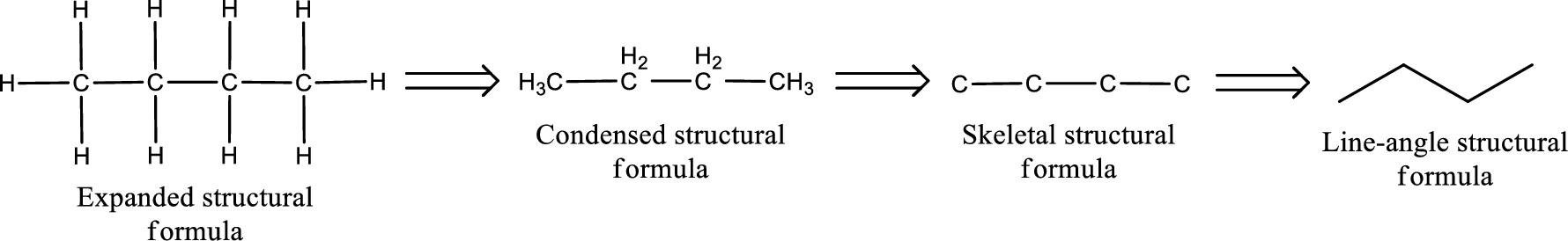Chapter 4, Problem 4.56EP### Organic And Biological Chemistry

7th Edition
STOKER + 1 other
ISBN: 9781305081079

#### Solutions

Chapter
Section### Organic And Biological Chemistry

7th Edition
STOKER + 1 other
ISBN: 9781305081079
Textbook Problem

# Draw skeletal structural formulas for the eight aldehydes and six ketones that have the molecular formula C6H12O.

Interpretation Introduction

Interpretation:

Skeletal structural formula three ketones and four aldehydes that have molecular formula of C6H12O has to be drawn.

Concept Introduction:

Isomers are the compounds that have same molecular formula but different structural formula.  The main difference lies in the way the atoms are arranged in the structure.  Isomers have different chemical and physical properties even when they have same molecular formula.  This is known as Isomerism.

If there is difference only in the connectivity of the atoms in the molecule, then it is known as constitutional isomerism.  The isomers are known as constitutional isomers.  They will have same molecular formula and same functional group, but they differ in the connectivity between the atoms in the molecule.

Aldehydes and ketones have constitutional isomers.  Functional group isomerism exists between aldehyde and ketones.  Aldehydes and ketones that have same degree of saturation and same number of carbon atoms are functional group isomers.  In aldehyde, skeletal isomerism is possible where the group attached to the carbonyl carbon atom connectivity is changed.  In ketones positional isomerism is possible where the carbonyl group is moved within the carbon chain.

The structural representation of organic compound can be done in 2D and 3D.  In two-dimensional representation, there are four types of representation in which an organic compound can be drawn.  They are,

• • Expanded structural formula
• • Condensed structural formula
• • Skeletal structural formula
• • Line-angle structural formula

Structural formula which shows all the atoms in a molecule along with all the bonds that is connecting the atoms present in the molecule is known as Expanded structural formula.

Structural formula in which grouping of atoms are done and in which the central atoms along with the other atoms are connected to them are treated as group is known as Condensed structural formula.

Structural formula that shows the bonding between carbon atoms alone in the molecule ignoring the hydrogen atoms being shown explicitly is known as Skeletal structural formula.

Structural formula where a line represent carbon‑carbon bond and the carbon atom is considered to be present in each point and the end of lines is known as Line-angle structural formula.Explanation

Given description in the problem statement reads that the compound has molecular formula of C6H12O.  For an aldehyde compound a minimum of one carbon is enough.  In the given molecular formula a total of six carbon atoms is present.  For an aldehyde, the terminal carbon atom has to be the carbonyl carbon atom.  The other five atoms can be arranged in various ways to obtain different aldehyde.  The skeletal structural formulas of the eight aldehydes with the molecular formula C6H12O is given below,

### Still sussing out bartleby?

Check out a sample textbook solution.

See a sample solution

#### The Solution to Your Study Problems

Bartleby provides explanations to thousands of textbook problems written by our experts, many with advanced degrees!

Get Started